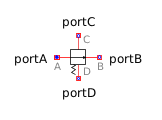Sum - MapleSim Help

Complex Sum

Output the sum of the elements of the input vectorDescription The Complex Sum (or Sum) component computes the scaled sum of the elements of the input signal vector u. A complex parameter value may be entered using the complex constructor, for example, Complex(3,4), or using I for the imaginary unit, 3+4*I. If a purely real value is entered, the real value will be converted to its complex equivalent.Equations $y={\sum }_{i=1}^{n}\phantom{\rule[-0.0ex]{5.0px}{0.0ex}}{k}_{i}{u}_{i}$Connections

 Name Description Modelica ID $u$ Complex input signals u $y$ Complex output signal yParameters

 Name Default Units Description Modelica ID $n$ $1$ Number of inputs nin $k$ $\mathrm{fill}\left(1,\mathrm{nin}\right)$ sum coefficients kModelica Standard Library The component described in this topic is from the Modelica Standard Library. To view the original documentation, which includes author and copyright information, click here.